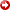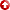[First Hit]Abstract:NonlinearInversion of the P-wave P-wave Reflection Coefficient Data

A. J. Pate

Surface seismic data are used to estimate lithologic parameters at an interface. The four unknown independent parameters at an interface are the ratio of the P-wave velocities and the ratio of the densities of upper and lower media, and the P-wave/S-wave velocity ratios in the upper and lower media respectively. The forward problem is solved by a reparameterized form of the full Zoeppritz equation for PP reflections. The inversion model is fitted to the data using a two part inversion scheme. The near offset (near normal incidence) data is initially inverted using a linearized Zoeppritz normal incidence equation to obtain estimates of the P-wave ratio and density ratio. The estimates of these two parameters are then used as initial guesses in anonlinearfull Zoeppritz in ersion by a Levenberg Marquardt procedure. Partial derivatives of the reparameterized Zoeppritz equation for the Jacobian matrix are calculated analytically at each iteration. All parameters are successfully estimated from synthetic data. Poisson's ratio of the upper and lower media can be calculated from inversion estimates of P-wave/S-wave velocity ratio. Lithologic parameters are estimated for several CDP gathers from a 3D survey of the Rabbit Hills Field in North Central Montana. A sensitivity analysis for the different parameters is performed.

AAPG Search and Discovery Article #90952©1996 AAPG Rocky Mountain Section Meeting, Billings, Montana